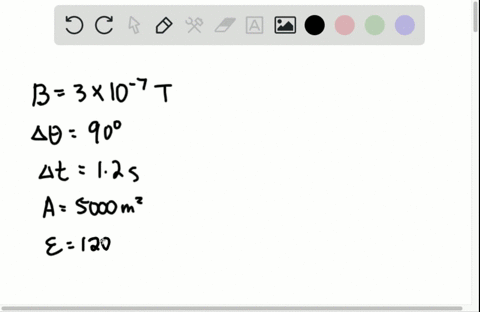🎉 The Study-to-Win Winning Ticket number has been announced! Go to your Tickets dashboard to see if you won! 🎉View Winning Ticket### Generator for space station Astronauts on a space…

03:14Indian Institute of Technology Kharagpur
Problem 35

# Equation Jeopardy 3 Invent a problem for which the fol- lowing equation might be a solution. $$\varepsilon=-(35) \frac{(0.12 \mathrm{T})\left(\cos 0^{\circ}\right)\left[(0)^{2}-\pi(0.10 \mathrm{m})^{2}\right]}{(3.0 \mathrm{s}-0)}$$

## Discussion

You must be signed in to discuss.

## Video Transcript

We know that induced electric field equals negative. She engine time on genuine flocks. So negative if we just used a delta notation flux is given by be dark A which is B a We'll sign Tetteh if we have constant magnetic field. Andi, the orientation off the magnetic field which respected the loop co sign data does not change. Then we can take that out off the different sign. Well, we would have delta a on down below. I have Delta Bi this particle That problem we have once again if there is Ah, if there's Ah look with with in the dance then he was having in the end is not changing. So in this particle that rob them induce electric field is given by negative 35 I'm sorry. Ah negative 35 times 0.1 Protester, go sign zero degree and I am going to write Eat like zero minus by times zero foreign 01 square divided by three seconds minus zero second. Now when can you have this problem? You can have this kind of situation where you can say that if there's a look with ready a 0.1 mater then by times 0.1 square becomes the area. So there is a change in area that is happening over here. Okay, so now you can think that there is, ah, 0.1 to test the off magnetic fields coming downwards. And you have Ah, some people. A loop over here which has got our radius R on our ease. 0.1 meter. The face off the face of the loop is perpendicular to the manager trilled within three seconds. We didn't three seconds. The the the loop Waas collapse to have zero area. So once again you have Ah, loop. You have a loop like this. Wheat in equals 35. There were 35 tons in the loop. It's a circular look with ready A 0.1 meter 0.1 Tesla magnetic fields This coming downward perpendicular to the face of the group. Within three second, you change the radius of the loop to zero. That is when you will have an electric field that will be given by these expression